PivotalR between R & PostgreSQL-like Databases(for exp : Greenplum, hadoop access by hawq)

+关注继续查看
PivotalR是R的一个包, 这个包提供了将R翻译成SQL语句的能力, 即对大数据进行挖掘的话. 用户将大数据存储在数据库中, 例如PostgreSQL , Greenplum.

PivotalR-package
An R font-end to PostgreSQL and Greenplum database, and wrapper
for in-database parallel and distributed machine learning open-source

Description
PivotalR is a package that enables users of R, the most popular open source statistical programming
language and environment to interact with the Pivotal (Greenplum) Database as well as Pivotal
HD/HAWQ for Big Data analytics. It does so by providing an interface to the operations on tables/views
in the database. These operations are almost the same as those of data.frame. Thus the
users of R do not need to learn SQL when they operate on the objects in the database. The latest
code is available at https://github.com/madlib-internal/PivotalR. A training video and a
quick-start guide are available at http://zimmeee.github.io/gp-r/#pivotalr.

Details
Package: PivotalR
Type: Package
Version: 0.1.17
Date: 2014-09-15
Depends: methods, DBI, RPostgreSQL

This package enables R users to easily develop, refine and deploy R scripts that leverage the parallelism
and scalability of the database as well as in-database analytics libraries to operate on big
data sets that would otherwise not fit in R memory - all this without having to learn SQL because
the package provides an interface that they are familiar with.

The package also provides a wrapper for MADlib. MADlib is an open-source library for scalable
in-database analytics. It provides data-parallel implementations of mathematical, statistical and
machine-learning algorithms for structured and unstructured data. The number of machine learning
algorithms that MADlib covers is quickly increasing.

As an R front-end to the PostgreSQL-like databases, this package minimizes the amount of data
transferred between the database and R. All the big data is stored in the database. The user enters
their familiar R syntax, and the package translates it into SQL queries and sends the SQL query into
database for parallel execution. The computation result, which is small (if it is as big as the original
data, what is the point of big data analytics?), is returned to R to the user.

On the other hand, this package also gives the usual SQL users the access of utilizing the powerful
analytics and graphics functionalities of R. Although the database itself has difficulty in plotting,
the result can be analyzed and presented beautifully with R.

This current version of PivotalR provides the core R infrastructure and data frame functions as well
as over 50 analytical functions in R that leverage in-database execution. These include

* Data Connectivity - db.connect, db.disconnect, db.Rquery
* Data Exploration - db.data.frame, subsets
* R language features - dim, names, min, max, nrow, ncol, summary etc
* Reorganization Functions - merge, by (group-by), samples
* Transformations - as.factor, null replacement
* Algorithms - linear regression and logistic regression wrappers for MADlib

Note
This package is differernt from PL/R, which is another way of using R with PostgreSQL-like
databases. PL/R enables the users to run R scripts from SQL. In the parallel Greenplum database,
one can use PL/R to implement parallel algorithms.

However, PL/R still requires non-trivial knowledge of SQL to use it effectively. It is mostly limited
to explicitly parallel jobs. And for the end user, it is still a SQL interface.

This package does not require any knowledge of SQL, and it works for both explicitly and implicitly
parallel jobs by employing the open-source MADlib library. It is much more scalable. And for the
end user, it is a pure R interface with the conventional R syntax.

Author(s)
Author: Predictive Analytics Team at Pivotal Inc. <user@madlib.net>, with contributions from
Data Scientist Team at Pivotal Inc.
Maintainer: Caleb Welton, Pivotal Inc. <cwelton@pivotal.io>

References

madlib.glm Linear, logistic and multinomial logistic regressions
madlib.summary summary of a table in the database.

Examples
## Not run:
## get the help for the package
help("PivotalR-package")
## get help for a function
## create multiple connections to different databases
db.connect(port = 5433) # connection 1, use default values for the parameters
db.connect(dbname = "test", user = "qianh1", password = "", host =
db.list() # list the info for all the connections
## list all tables/views that has "ornst" in the name
db.objects("ornst")
## list all tables/views
db.objects(conn.id = 1)
## create a table and the R object pointing to the table
## using the example data that comes with this package
delete("abalone", conn.id = cid)
x <- as.db.data.frame(abalone, "abalone")
## OR if the table already exists, you can create the wrapper directly
## x <- db.data.frame("abalone")
dim(x) # dimension of the data table
names(x) # column names of the data table
madlib.summary(x) # look at a summary for each column
lk(x, 20) # look at a sample of the data
## look at a sample sorted by id column
lookat(sort(x, decreasing = FALSE, x$id), 20) lookat(sort(x, FALSE, NULL), 20) # look at a sample ordered randomly ## linear regression Examples -------- ## fit one different model to each group of data with the same sex fit1 <- madlib.lm(rings ~ . - id | sex, data = x) fit1 # view the result lookat(mean((x$rings - predict(fit1, x))^2)) # mean square error
## plot the predicted values v.s. the true values
ap <- x$rings # true values ap$pred <- predict(fit1, x) # add a column which is the predicted values
## If the data set is very big, you do not want to load all the
## data points into R and plot. We can just plot a random sample.
random.sample <- lk(sort(ap, FALSE, "random"), 1000) # sort randomly
plot(random.sample) # plot a random sample
## fit a single model to all data treating sex as a categorical variable ---------
y <- x # make a copy, y is now a db.data.frame object
y$sex <- as.factor(y$sex) # y becomes a db.Rquery object now
fit2 <- madlib.lm(rings ~ . - id, data = y)
fit2 # view the result
lookat(mean((y$rings - predict(fit2, y))^2)) # mean square error ## logistic regression Examples -------- ## fit one different model to each group of data with the same sex fit3 <- madlib.glm(rings < 10 ~ . - id | sex, data = x, family = "binomial") fit3 # view the result ## the percentage of correct prediction lookat(mean((x$rings < 10) == predict(fit3, x)))
## fit a single model to all data treating sex as a categorical variable ----------
y <- x # make a copy, y is now a db.data.frame object
y$sex <- as.factor(y$sex) # y becomes a db.Rquery object now
fit4 <- madlib.glm(rings < 10 ~ . - id, data = y, family = "binomial")
fit4 # view the result
## the percentage of correct prediction
lookat(mean((y$rings < 10) == predict(fit4, y))) ## Group by Examples -------- ## mean value of each column except the "id" column lk(by(x[,-1], x$sex, mean))
## standard deviation of each column except the "id" column
lookat(by(x[,-1], x$sex, sd)) ## Merge Examples -------- ## create two objects with different rows and columns key(x) <- "id" y <- x[1:300, 1:6] z <- x[201:400, c(1,2,4,5)] ## get 100 rows m <- merge(y, z, by = c("id", "sex")) lookat(m, 20) ## operator Examples -------- y <- x$length + x$height + 2.3 z <- x$length * x\$height / 3
lk(y < z, 20)
## ------------------------------------------------------------------------
## Deal with NULL values
delete("null_data")
x <- as.db.data.frame(null.data, "null_data")
## OR if the table already exists, you can create the wrapper directly
## x <- db.data.frame("null_data")
dim(x)
names(x)
## ERROR, because of NULL values
fit <- madlib.lm(sf_mrtg_pct_assets ~ ., data = x)
## remove NULL values
y <- x # make a copy
for (i in 1:10) y <- y[!is.na(y[i]),]
dim(y)
fit <- madlib.lm(sf_mrtg_pct_assets ~ ., data = y)
fit
## Or we can replace all NULL values
x[is.na(x)] <- 45
## End(Not run)

> install.packages("PivotalR")
> library(PivotalR)
Attaching package: ‘PivotalR’
The following objects are masked from ‘package:stats’:
sd, var
The following object is masked from ‘package:base’:
cbind

[参考]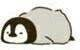9 08 0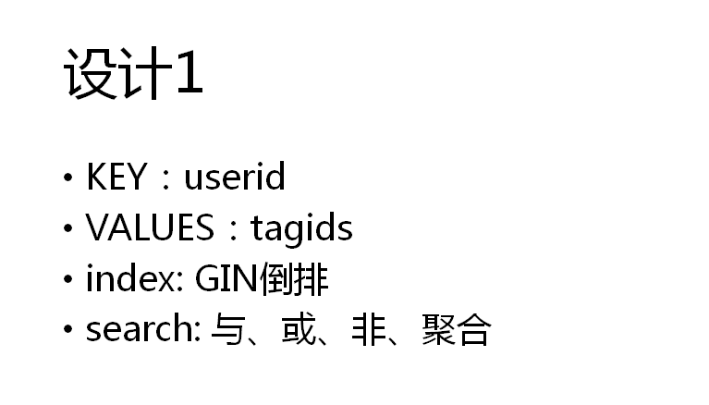PG+MySQL第9课-实时精准营销

6 0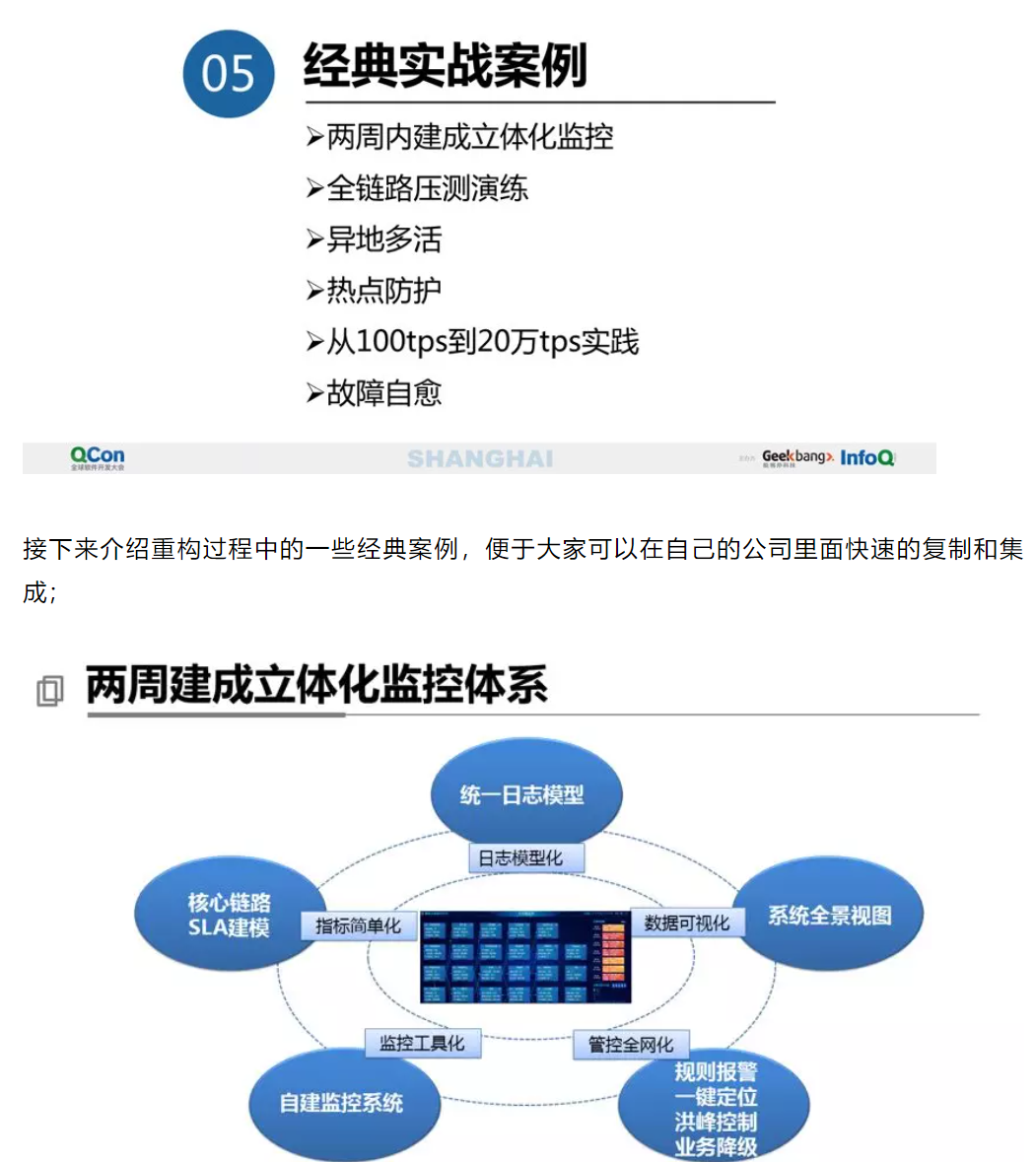【技术干货】40页PPT分享万亿级交易量下的支付平台设计（6）
【技术干货】40页PPT分享万亿级交易量下的支付平台设计（6）
8 0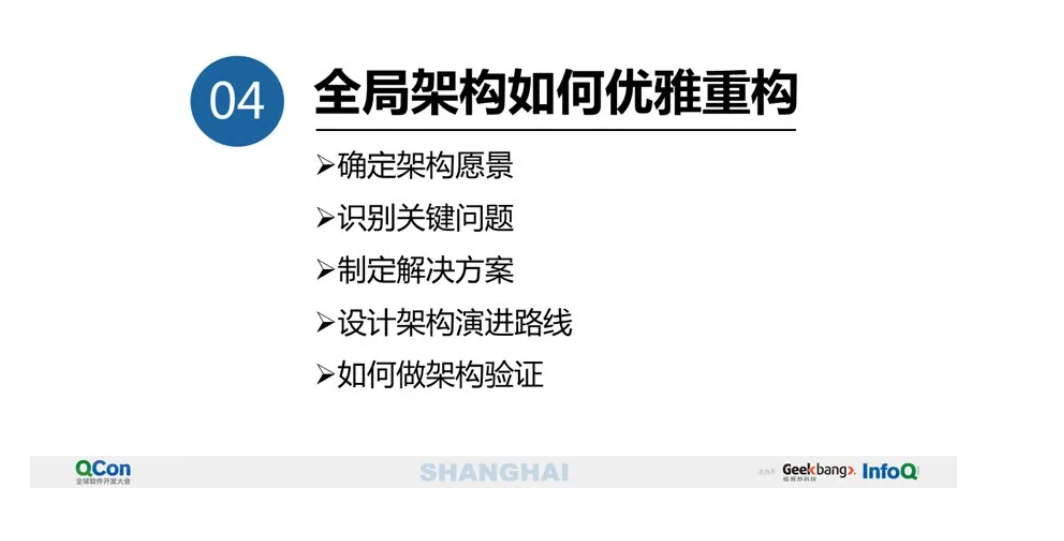【技术干货】40页PPT分享万亿级交易量下的支付平台设计（4）
【技术干货】40页PPT分享万亿级交易量下的支付平台设计（4）
5 0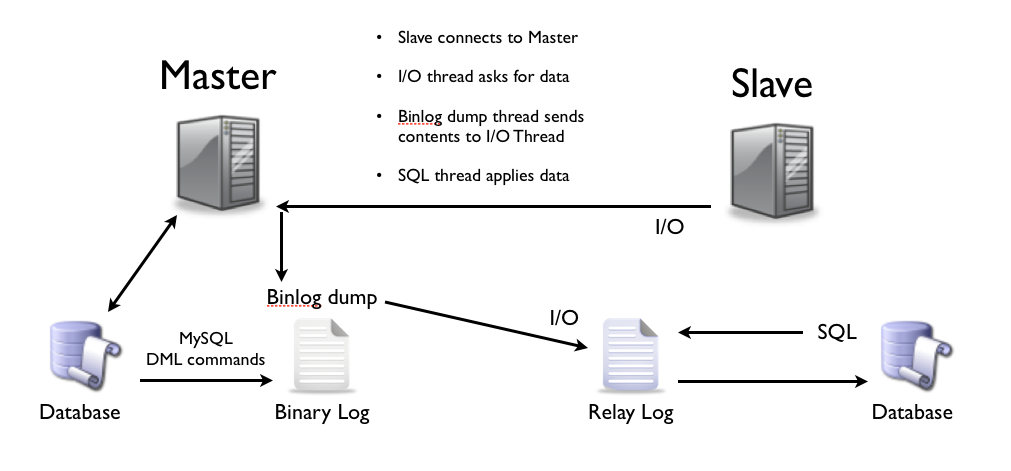MySQL高可用架构演进
MySQL是数据库领域当之无愧的霸主之一，其在各行各业被广泛应用，随着广泛使用，对于MySQL本身的高可用性的要求就是不可避免的话题，而MySQL的高可用方案也随着MySQL功能的完善经历了多次升级，本文将对MySQL的各种高可用架构进行分析，以此来了解架构的演进。
7 0+关注

2153

245

《2021云上架构与运维峰会演讲合集》

《零基础CSS入门教程》

《零基础HTML入门教程》